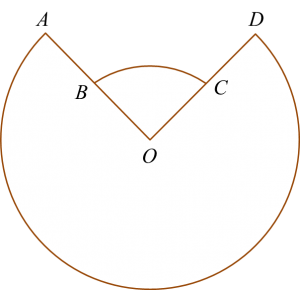\

# SPM Additional Mathematics 2017, Paper 1 (Question 14 – 16)

Question 15 (4 marks):
It is given that cos α = t where t is a constant and 0o ≤ α ≤ 90o.
Express in terms of t
(a) sin (180o + α),
(b) sec 2α.

Solution:
(a)$\begin{array}{l}\text{sin}\left({180}^{o}+\alpha \right)\\ =\mathrm{sin}180\mathrm{cos}\alpha +\mathrm{cos}180\mathrm{sin}\alpha \\ =0-\mathrm{sin}\alpha \\ =-\mathrm{sin}\alpha \\ =-\sqrt{1-{t}^{2}}\end{array}$

(b)

Question 16 (3 marks):
Diagram 7 shows two sectors AOD and BOC of two concentric circles with centre O.Diagram 7

The angle subtended at the centre O by the major arc AD is 7α radians and the perimeter of the whole diagram is 50 cm.
Given OB = r cm, OA = 2OB and ∠BOC = 2α, express r in terms of α.

Solution: